Math Concepts

# How to find the best fit line?

1.2k views

 1 Introduction 2 Types of Data 3 Introduction to Graphical Data & Line of Best Fit 4 Paper & Pencil Solution 5 Least Square Method 6 Conclusion 7 FAQs

11 December 2020

## Introduction

Recently, we may have come across various types of graphs of COVID-19 data.

How do they predict the extent of disease?

That’s when the best fit line comes into the picture. The best fit line is also called a regression line.

The line of best fit is a line that best represents the relationship between a scatter plot of data points.

Just by looking at the data points, we can’t predict anything.

Whereas, if we use these data points to create the best fit line, we can easily predict the future values. Download the PDF below to understand the best fit line.

## Types of Data

### Discrete Data

• Discrete data can only take certain values.
• It can be counted.
• It cannot be divided.
• It can be graphed on a bar graph.
• Example: number of dogs, number of books, number of students, etc.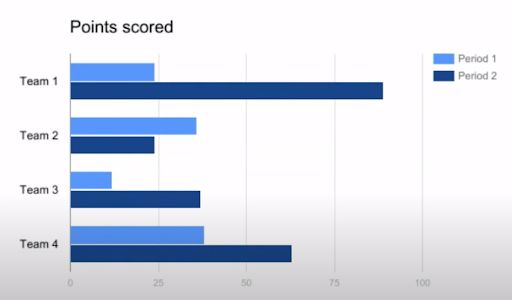### Continuous Data

• Continuous data can take any value within a range.
• It can be measured.
• It can be divided.
• It can be graphed on a histogram or a line graph.
• Example: time, the weight of a dog, the height of the students etc.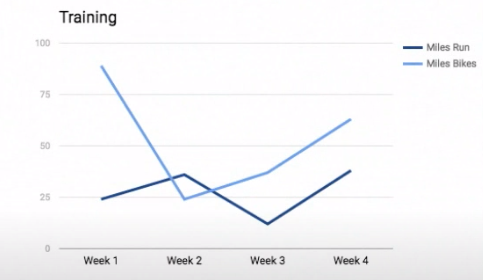## Introduction to Graphical Data & Line of Best Fit

Various charts and graphs can be used to visually display and analyze the graphical data.

It helps us to quickly highlight the behaviour and relationship between data points which may contribute to better understanding and predictions.

Depending on the type of data we may use a bar graph, histogram, line graph, heat map, Venn diagram, pie chart, etc.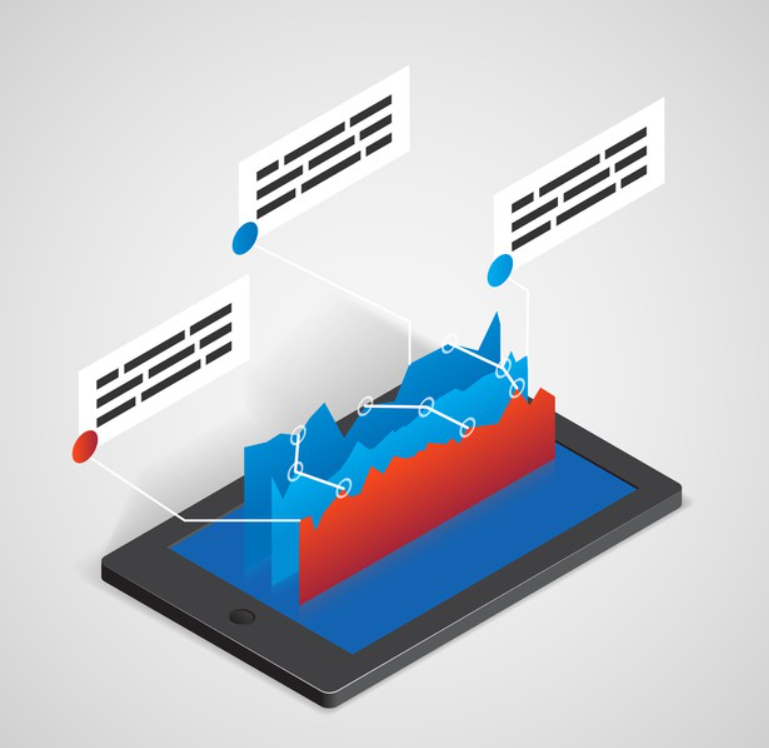Line of best fit refers to a line that best expresses the relationship between a scatter plot of data points.

Statisticians generally use the least-squares method to find the equation of the best fit line.

### Various types of best fit line are-

• Linear Best Fit Line: The line of best fit is a straight line when data points appear to be in a straight line.
• Polynomial Best Fit Line: A polynomial best fit line has many curves and bumps. In the real-world, data usually follows a polynomial trend.
• Exponential Best Fit Line: An exponential best fit line shows exponential growth. Eg: rise in COVID-19 cases etc.## Paper and Pencil Solution

The best fit line may or may not pass through all the given data points. Paper & Pencil Solution is one of the methods to find the line of best fit.

1. Prepare a scatter plot of the data points on the graph sheet.
2. Using a thread, position it such that the plotted points are as close to the thread as possible.
3. Mark the two points which you think would be included in the line of best fit.
4. Calculate the slope through these two points.
5. Write the equation of the line passing through the two points. This equation will be the line of best fit for the give data points.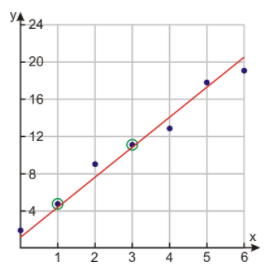## Least Square Method

The least-square method is a more accurate way of finding the line of best fit. Use the following steps to find out the equation of the best fit line for a set of data points (x1,y1),(x2,y2)...(xn,yn).

1. Calculate the mean of x values and y values using the formula: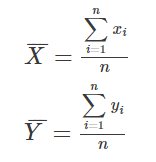2. Find the slope of the best fit line using the formula: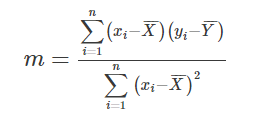3. Find the y-intercept of the line using the formula: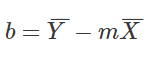4. Write the equation of the best fit line using slope and the y-intercept.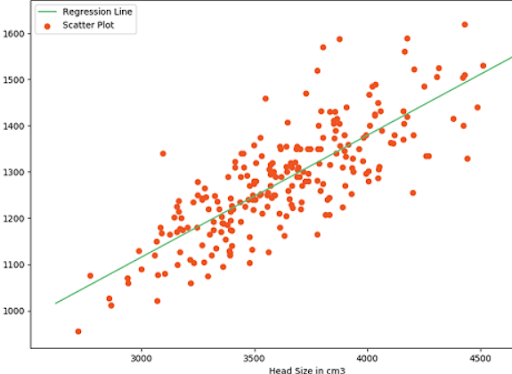## Conclusion

The least Square method is the most popular method to find the best fit line.

Make sure to practice it more until you feel confident about this topic as it is used heavily in future classes.

Did you know that this best fit line is the core concept of the Machine learning algorithm called Linear Regression?

If not, now you know the basic concept of one ML algorithm as well, make sure to tell your friends that!

Cuemath, student-friendly mathematics and coding platform, conducts regular Online Live Classes for academics and skill-development, and their Mental Math App, on both iOS and Android, is a one-stop solution for kids to develop multiple skills.

Check out the fee structure for all grades and book a trial class today!

## What is data?

Data is a collection of facts and statistics for analysis.

## How do you differentiate between data and information?

Data is a collection of facts. Whereas, information is how you understand those facts.

## What are the ways to represent data?

Data can be represented using graphs, tables and charts.

## What are the different types of graphs?

Various types of graphs are:

• bar graph
• line graph
• pictograph etc.

## What is best fit line?

The best fit line is a line which best represents the relationship between a scatter plot of data points.

## What is meant by line fitting?

Line fitting is the process of constructing a best fit line.

## What is the use of best fit line?

The best fit line helps us to predict future values.

## External References

Related Articles
Award-winning math curriculum, FREE for a year